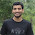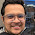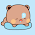Wednesday, July 8, 2009

Factorial of negative number

Is it possible to find factorial of negative numbers?

By definition,
N! = 1 x 2 x .... x N
⇒ N! = 1 x 2 x .... x N x (N+1) / (N+1)
⇒ N! = (N+1)! / (N+!)

Now, let's try some values:
3! = 4! / 4 = 6
2! = 3! / 3 = 2
1! = 2! / 2 = 1
0! = 1! / 1 = 1

So, that's how 0! = 1. Let's not stop at 0!, if we continue this fashion, we get:
(-1)! = 0! / 0 = Undetermined

Many people still keeps arguing about whether x / 0 = +∞ or Undefined, it really doesn't matter though, because it is well established that anything divided by zero is undefined.

However, in this post, we are going to talk about a problem from UVa Online Judge, UVa-10323 (Factorial! You Must be Kidding!!!). This problem actually requires you to calculate even the factorials of negative numbers, which does not really exist in real world.

In this context, we are forced to assume that x / 0 = +∞. There was a mistake on my previous post, this section is updated as per the comment from a fellow reader আহনাফ তাহমিদ:
(-1)! = 0! / 0 = +∞
(-2)! = (-1)! / -1 = -∞
(-3)! = (-2)! / -2 = +∞
(-4)! = (-3)! / -3 = -∞
(-5)! = (-4)! / -4 = +∞
and so on..

Hence, we can see that, if N is odd, then (-N)! = +∞, otherwise (-N)! = -∞. Note that, this specific problem statement calls +∞ as Overflow and -∞ as Underflow.

Hope it helps!

1.Yeap.... Very nice SVI or SVI or Sir-Vaiya...

2.Good Explenation..! Thank you

3.good way of thinking....even if i do not share your opinion about dividing by zero.

4.It is well defined that dividing by zero is undefined, it is pointless to argue about that.

5.some where i have seen (-1)!=0, but i have no idea

6.Great Explanation!

Thanks
Anjaneai

7.Yah, thanks to wikipedia man!

8.ওকে ভাইয়া, আমার মনে হয় এইটা একটু গোলমাল হচ্ছেঃ
if n<0
(n%2==0)
n!=-infinity
else
n!=+infinity
আমার হিসেবে এইটা আসছে।
কারণ আমরা যখন (-1)! -হিসাব করছি তখন (-১)! = ০!/০ = INFIN
আবার (-২)! = (-১)!/(-১) যেহেতু (-১)! = INFIN তাই ব্যাপারটা দাঁড়াচ্ছে INFIN/ (-১)= -INFIN। এখন ২ যেহেতু জোড় তাই জোড়ের জন্য -INFIN।
আবার, (-৩)! = (-২)!/(-২)=-INFIN/-২ = INFIN। কাজেই, বিজোড় হলে +INFIN।
আমার ধারণায় ভুল থাকতে পারে ভুল পেলে জানাবেন।

1.You assumed 0/0 = +inf, why not -inf?

9.তাহলে UVA এর প্রবলেমটাতে
if (num<0 && num%2==1) printf("Overflow!\n");
else if (num<0 && num%2==0) printf("Underflow!\n");

এই ক্ষেত্রে শুধু রাইট আনসার দিচ্ছে কেন?

1.umm, ok, I will update this section properly, thanks.
but still, dividing anything by 0 doesn't make any sense, only Shahriar Manzoor can do stuffs like that.

2.Post updated, thanks again :)

10.না ভাইয়া, ধন্যবাদ আপনাদের। যারা এত কষ্ট করে আমাদের জন্য এত কিছু করেন।
#রেস্পেক্ট।

11.can anyone give me more link of problem to use this technique??

12.Very informative article.Thank you author for posting this kind of article .

http://www.wikitechy.com/view-article/c-program-to-find-factorial-of-a-number-with-example-and-explanation

Both are really good,
Cheers,
Venkat